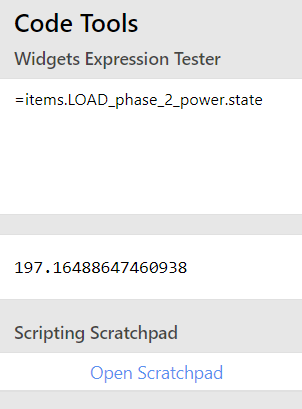# Logical operation in the widget is not working

Dear,
I’m perplexed by the behaviour of one widget.

The color of the value is conditional and in all 3 items the code is exactly the same

``````color: '=items.LOAD_phase_1_power.state > items.LOAD_phase_limit.state ? "red" :  "gray"'

``````

just with the difference of the phase number. HOW COME the phase2 condition comes up true???The conditions are wrong also in the scrachpad.

That is impossible. if I play in the limit slider I noticed that it this condition changes to false when `LOAD_phase_limit.state` is 2000 as if the` LOAD_phase_2_power.state` is taken into this condition multipied by 10, but then when set to 600 is evaluated false for all 3 phases so in that moment phase_1 and phase_3 is wrong. so it is random.

TIA
Maciej

AFAIK `state` is a string therefore operators as `>` will not give expected results. Try something like

`Number.parseFloat(items.LOAD_phase_1_power.state) > Number.parseFloat(items.LOAD_phase_limit.state) `

Answering from my phone so syntax might not be 100% correct but you should get the idea …

2 Likes

`state` is always a string in the widgets, so you are doing a string comparison not a numerical comparison. You want:

``````color: '=Number(items.LOAD_phase_1_power.state) > Number(items.LOAD_phase_limit.state) ? "red" :  "gray"'
``````
2 Likes

Allow me to add - there is a small difference between the answers:

`parseFloat` will only parse an input up to the point where it stops making sense, and return that number. For example, numbers with units after the number can be parsed because the number comes before the unit:

``````Number.parseFloat("3px"); // => 3
Number.parseFloat("9,59 €"); // => 9
``````

On the other hand, `Number` will try to parse the whole value as a number, including any text:

``````Number("3px"); // => NaN
Number("9,59 €"); // => NaN
``````

This will come into play when you have a quantity associated with `state`, i.e. `30 °C` where

``````Number.parseFloat("30 °C") // => 30
``````

while

``````Number("30 °C") // => NaN
``````

so the `Number.parseFloat` might be a bit “safer”. More details here.

EDIT: Please note, while `Number.parseFloat("30 °C")` will be able to parse numerical value from string, it will not do any conversion, so

``````Number.parseFloat("30 °C") == Number.parseFloat("30 °F") // => true !!!
``````
2 Likes

Many thanks guys ! fixed it in a blink of an eye.